Disclaimer: This is an example of a student written essay.

Any scientific information contained within this essay should not be treated as fact, this content is to be used for educational purposes only and may contain factual inaccuracies or be out of date.

# Velocity of Sound Experiment

 ✅ Paper Type: Free Essay ✅ Subject: Physics ✅ Wordcount: 2968 words ✅ Published: 9th Mar 2018

Experiment no. 7: Velocity of Sound

Laboratory Report

Von Dela Rosa, Anna De Vera, Tricia Desierto,

Department of Biological Science

College of Science, University of Santo Tomas

EspanÌƒa, Manila, Philippines

Abstract

Three activities were done in the experiment to demonstrate and further analyze the velocity of sound. In the first activity, the velocity of sound in air and in glass tube was computed. In the second activity, the speed of sound was computed using vernier microphone connected to vernier logger pro. Lastly, the speed of sound in solid using Kundt’s tube was calculated. In the data, it has been found out that the speed of sound in air has a theoretical speed of 347.8 m/s. A percent error of 7.4%, and 10.16% were acquired in the first experiment using different frequencies, and 5.66% error was acquired in the second activity. Lastly, it has been found out that the speed of sound in solid rod is computed to be 5044.33 m/s with a percent error of 15.33% when done experimentally.

I. Introduction

The pressure disturbance travelling from one particle to another connotes to the velocity of sound. Basically, a sound wave is a travelling disturbance. Wave pulse connotes to a single disturbance while wave train is the series of disturbances. Wave frequency measures the number of pulse have been made in a length of given time. In the simplest sense, frequency is the number of vibrations per seconds. It is usually measured in Hertz(Hz). On the other hand, resonance is the natural vibration frequency of an object. In the experiment, three activities have been done in order to exemplify the different properties of sound.

The objectives of the experiment are:

a.) to verify the relationship between frequency of sound and its wavelength

b.) to determine the speed of sound by means of a resonating air column; and

c.) to determine the velocity of sound in a solid using a vibrating rod.

II. Theory

Sound wave

Sound wave is a disturbance that travels from one location to another location. It is propagated by vibrating objects and can be transmitted through liquid, plasma, or gases as a longitudinal waves though in solids, sound waves can be transmitted as a longitudinal wave or transverse wave. Longitudinal wave is a kind of wave wherein the particles move in a parallel direction relative to the wave direction. On the other hand, transverse wave vibrate perpendicular to the direction of wave motion.

Frequency

Frequency is defined as the number of waves per unit of time. Frequency can be computed by dividing the velocity of sound in air to the twice the mean distance of two nodes.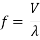V= Theoretical Value of the speed of sound in air

ðœ†= Twice the average of the distances between two consecutive displacement nodes

Equation 1. Frequency of a Sound

Resonating Air Column

The basic principle behind the cylindrical air column is that resonance waves will only be produced at a definite frequency. The closed end of the column depicts the wave node and the open end is the antinode. If the frequency of the column is equal to the frequency of the tuning fork, achieving resonance, the intensity of the sound is increased. Therefore, if the frequency of the tuning fork is not equal to the frequency of the column, resonance will not occur and the sound will be faint.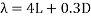L=distance between a point and the top of the glass tube

D= Diameter of the resonance tube

Equation 2. Equation for wavelength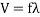f= Frequency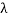= wavelength

Equation 3. Equation for Velocity of sound in air inside the glass tube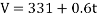t= Temperature

Equation 4. Equation for speed of sound in air at a certain temperature

### Kundt’s tube apparatus

Kundt’s tube is an apparatus allows the group to calculate the speed of sound. Sound waves can travel to the tube by rubbing the metal end which will create a squeaking sound. In that scenario, the vibration of the rod will vibrate the disk causing it to propagate the frequency of the rod to the glass tube. Thus, the air inside the tube will move in response to the sound waves. Fine sand will take note of the air’s response to the sound wave by exposing the striations done by rubbing the metal end. The antinode, and the node were then noted. The wavelength of sound is twice the length of the rod. Equation for the speed of sound in the rod is shown in Equation5. The Equation for the theoretical speed of sound in the rod is shown in Equation 6.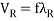f= FrequencyR= Wavelength of sound in the rod

Equation5.Speed of sound in solid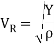Y= Young’s modulus

ρ= Density of the rod

Equation 6. Theoretical speed of sound in the rod

III. Methodology

The materials used are resonance tube apparatus, two tuning forks with different frequencies, meter stick, rubber mallet, thermometer, and Kundt’s tube apparatus.

Activity 1: Resonating Air Column

Starting with the water near the top of the resonance tube apparatus, the group had strike the tuning fork using a rubber mallet and placed it on top of the glass tube. The water level was lowered slowly until the loudest sound was heard. The water level was marked at the highest sound heard. After that, the distance between that point and the top of glass tube was measured. Also, the diameter of the resonance tube was also measured. The wavelength of the sound produced was calculated. Two trials have been done to determine the average wavelength. Using the average wavelength and frequency engraved, the velocity of the sound in air was computed. The temperature of air inside the glass tube was also determined without the thermometer touching the water. The speed of sound in air was computed using the noted temperature. The percent error was computed by comparing the speed using the average wavelength and frequency and the speed calculated using the temperature inside the tube. The procedure was computed using other tuning forks.

Activity 2: Speed of Sound

The vernier microphone was connected to Channel 1 of the interface. The position of the microphone was placed near the open end of a closed tube. The file “24 Speed of Sound” in Physics was opened. As soon as the data collection begins, the fingers were snapped near the tube. From the graph in the computer screen, te time interval between the start of the first vibration and start of echo was determined. That time was noted as the time interval for the sound to travel through tube and back. The speed of sound was computed by dividing the length of the tube by one-half of the time interval obtained from the graph. The percent error was computed with the accepted value obtained in the first activity.

Activity 3: Speed of Sound in Solid

A thin layer of cork dust was placed uniformly in the Kundt’s tube. The rod was clamped at the center. The rod was rubbed with a piece of cloth with coarse powder, setting the rod into vibration producing high frequency. The wave pattern will be formed in the cork dust inside the glass tube. The displacement of the two consecutive displacement nodes has been measured and the mean distanced of the distance was calculated. The frequency of the sound was also calculated. The speed of sound in the rod and the theoretical speed of sound in the rod were also computed. Lastly, the percent error was calculated using the computed data.

VI. Results and Discussion

The three activities that were done in this experiment elaborating the speed of sound as it travels along a path.

Activity 1: Resonating Air Column

Temperature of air: 280C

Diameter of Resonance Tube= 0.0344 m

 Frequency of Tuning Fork Wavelength (m) Trial 1 Trial 2 Trial 3 320 E 0.20m 0.25m 0.30m 512 C 0.10m 0.15m 0.20m

Table 1A: Measurements of Wavelengths

Table 1A shows the frequency of the Tuning Fork and the different wavelengths of the three trials in meters. The result of the wavelength of each trials means that these are the wavelengths where the members heard the loudest sound that the Tuning Fork produced.

 Ave. wavelength Exp. Speed Theor. Speed % error 0.25m 323.30m/s 347.8m/s 7.4% 0.15m 312.48m/s 347.8m/s 10.16%

Table 1B: Calculated Quantities

Table 1B shows the computed Average Wavelengths in unit of meters; the Experimental and Theoretical Speeds both in unit of meters per second; and their respective % errors.

Activity 2: Speed of Sound

 Length of Tube: 0.95m Total Travel Time Trial 1 0.0051s Trial 2 0.0058s Average 0.00545s Experimental Speed 361.47m/s Theoretical speed 347.8m/s % error 5.66 %

Table 2: Speed of Sound

Table 2 shows the Total and Average Travel Times of both trials in unit of seconds; the Experimental and Theoretical Speeds that were calculated in unit of meters per second; and the computed % error of 5.66%.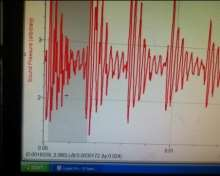Figure 1: Trial 1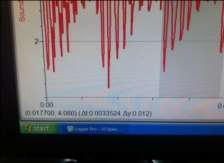Figure 2: Trial 2

Figures 1 and 2 shows the different

Time Interval of trials 1 and 2, between the

start of the first vibration and the start of the

echo vibration.

Activity 3: Speed of Sound in Solid

 Ave. of distances between node to node 0.055m Wavelength of sound in air 0.11m/s Frequency of sound 3161.81 Hz Length of rod 0.92m Wavelength of sound in rod 1.84m Exp. Speed of sound in the rod 5817.74m/s Theor. Speed of sound in rod 5044.33m/s % error 15.33%

Table 3: Speed of Sound in Solid

Table 3 shows the Average distances between node to node in meters; the wavelength of sound in air in meters per second; the frequency of sound in hertz; the length of the rod and the wavelength of sound in rod both in unit of meters, the wavelength of sound in the rod is twice the length of the rod; the experimental and theoretical speeds both in meters per second; and the computed % error of 15.33%.

V. Conclusion

The relationship between the frequency of sound and its wavelength was verified. In conclusion, the frequency and wavelength is inversely proportional to one another. The speed of sound was successfully determined by means of a resonating air column with percent errors of 7.4% and 10.16%. The determination of velocity of sound in a solid was successful by using a vibrating rod with a percent error of 15.33%. It can therefore be concluded that the distance between node to node is equivalent to the wavelength of sound.

VI. Applications

1. What is the relation between frequency and wavelength of sound produced in a medium?

The frequency and wavelength is inversely proportional to one another. The longer the wavelength, the shorter the frequency and vice versa.

2. What is the use of water in the activity 1?

The water serves as reflector of sound waves back to the open end to cause a hearing sound if the frequency of the column equals to the frequency of the tuning fork.

3. In medical practice, ultrasound in the range of 1 to 5 megahertz is being used as an imaging modality, The associated wavelength in a typical human tissue range from 0.3mm to 0.06 mm. Find the velocity of ultrasound in the tissue.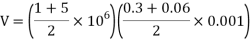4.The outer ear of a human may be thought of as closed pipe 2.7 cm long on the average. What frequency would be most effectively detected by the ear at 30 degrees Celsius.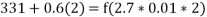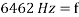5. Suppose that we increase the temperature of the air through which a sound wave is travelling, what effect does this have on the velocity of the wave. For a given frequency, what effect does increasing the temperature have on the wavelength of the sound wave? Explain.

If the temperature increases, then the molecules are being excited. Therefore the molecules vibrate faster, causing the velocity of the wave to travel faster. Increasing the temperature corresponds to shorter sound wave.

6. If you were lying on the ground, would you hear footsteps sooner or later with your ear touching the ground or not?

You would hear the footsteps sooner, because sound travels faster in a solid medium than in air.

References:

 Pitch and frequency. (n.d.). Retrieved May 9, 2014, from Physicsclassroom: http://www.physicsclassroom.com/class/sound/Lesson-2/Pitch-and-Frequency

  Resonant air column. (n.d.). Retrieved May 9, 2014, 2014, from hartnell: http://www.hartnell.edu/physics/labs/4c/2resonantaircolumn.pdf

 Speed Of Sound In Thin Metal Rods And Young Modulus. (n.d.). Retrieved May 9, 2014, from dsu.nodak: http://www2.dsu.nodak.edu/users/edkluk/public_html/nslab/ls_sv_ym.html

View all

## DMCA / Removal Request

If you are the original writer of this essay and no longer wish to have your work published on UKEssays.com then please:

Related Services

Prices from

\$USD152

Approximate costs for:

• 1000 words
• 7 day deliveryHumanity University

Dedicated to your worth and value as a human being!

Related Lectures